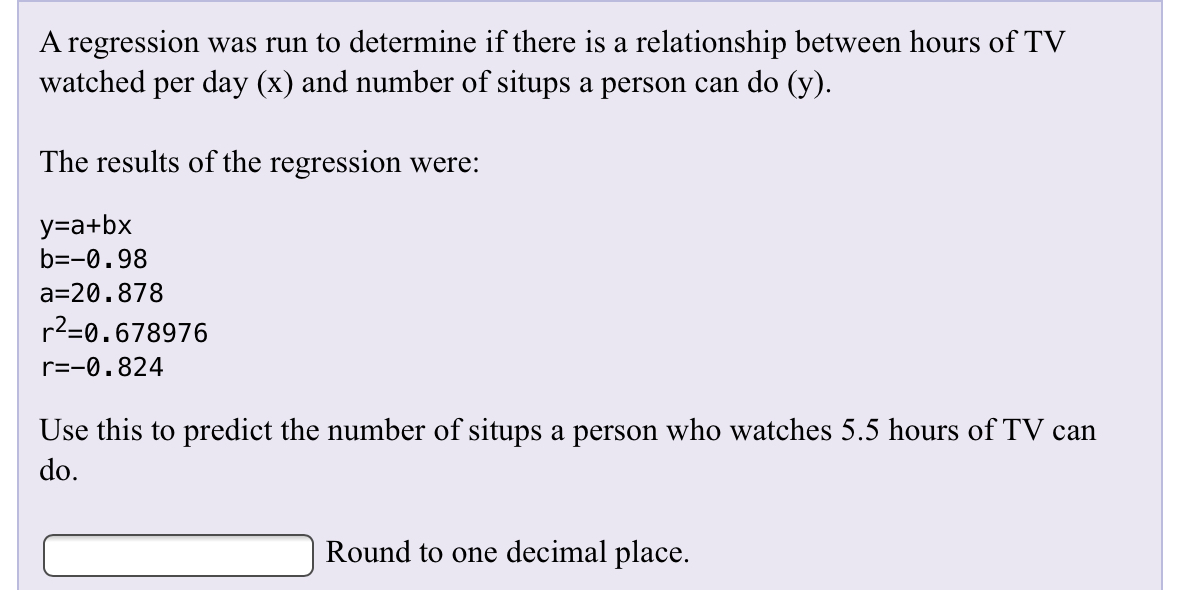# A regression was run to determine if there is a relationship between hours of TVwatched per day (x) and number of situps a person can do (y)The results of the regression were:у-а+bxb-0.98a-20.878r2-0.678976r=-0.824Use this to predict the number of situps a person who watches 5.5 hours of TV cando.Round to one decimal place.

Question
2 views

How do find the answer on paper and the TI84help_outlineImage TranscriptioncloseA regression was run to determine if there is a relationship between hours of TV watched per day (x) and number of situps a person can do (y) The results of the regression were: у-а+bx b-0.98 a-20.878 r2-0.678976 r=-0.824 Use this to predict the number of situps a person who watches 5.5 hours of TV can do. Round to one decimal place. fullscreen
check_circle

Step 1

Given data
y = a + bx
b = - 0.98
a = 20.878
r2 = 0.678976
r = -0.824
The least square regression equation is given by
y = a + bx
y = 20.878 + (-0.98) x
No of situps a person who watches 5.5 hours of tv is given by
putting x = 5.5 in least square regression equation
y = 20.878 + (-0.98) * 5.5
y = 15.488

Step 2

Using TI84

...help_outlineImage TranscriptioncloseTI-B3 Plus TEXAS INSTRUMENTS 20.878-0.98*5.5 15.488 MATPLOT EST ta CIC TLE Y WINDOR TRACE GRAPH ZOOM QUIT IND 2nd MODE DEL LIST A-LOCK LINK X38n ALPHA STAT ANGLE DRAW DISTR TEST MATH APPS PRGM VARS CLEAR MATRX SIN x COs TAN SIN COS TAN 10 LOG L4 LD LN L1 LS ACL MEM STO CATA ANS OFF ON ENTER fullscreen

### Want to see the full answer?

See Solution

#### Want to see this answer and more?

Solutions are written by subject experts who are available 24/7. Questions are typically answered within 1 hour.*

See Solution
*Response times may vary by subject and question.
Tagged in

### Other